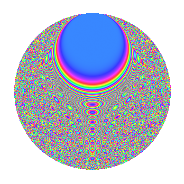# Properties

 Label 693.2.czLevel 693 Weight 2 Character orbit cz Rep. character $$\chi_{693}(40,\cdot)$$ Character field $$\Q(\zeta_{30})$$ Dimension 736 Newform subspaces 1 Sturm bound 192 Trace bound 0

# Related objects

## Defining parameters

 Level: $$N$$ = $$693 = 3^{2} \cdot 7 \cdot 11$$ Weight: $$k$$ = $$2$$ Character orbit: $$[\chi]$$ = 693.cz (of order $$30$$ and degree $$8$$) Character conductor: $$\operatorname{cond}(\chi)$$ = $$693$$ Character field: $$\Q(\zeta_{30})$$ Newform subspaces: $$1$$ Sturm bound: $$192$$ Trace bound: $$0$$

## Dimensions

The following table gives the dimensions of various subspaces of $$M_{2}(693, [\chi])$$.

Total New Old
Modular forms 800 800 0
Cusp forms 736 736 0
Eisenstein series 64 64 0

## Trace form

 $$736q - 10q^{2} - 9q^{3} + 170q^{4} - 9q^{5} - 5q^{7} - 40q^{8} - 9q^{9} + O(q^{10})$$ $$736q - 10q^{2} - 9q^{3} + 170q^{4} - 9q^{5} - 5q^{7} - 40q^{8} - 9q^{9} + 2q^{11} - 36q^{12} - 19q^{14} - 24q^{15} - 174q^{16} - 30q^{17} - 5q^{18} - 30q^{19} + 66q^{20} - 12q^{22} + 2q^{23} - 15q^{24} - 73q^{25} - 66q^{26} - 27q^{27} - 20q^{28} - 10q^{29} + 25q^{30} - 12q^{33} - 12q^{34} + 5q^{35} - 44q^{36} - 6q^{37} + 21q^{38} - 45q^{39} - 15q^{40} + 90q^{41} + 9q^{42} - q^{44} + 24q^{45} - 30q^{46} - 30q^{48} - 3q^{49} + 15q^{50} - 85q^{51} - 15q^{52} - 38q^{53} - 18q^{56} - 60q^{57} + 13q^{58} - 147q^{60} + 85q^{63} + 132q^{64} + 3q^{66} - 16q^{67} - 75q^{68} + 27q^{69} + 43q^{70} - 40q^{71} + 115q^{72} - 30q^{73} + 145q^{74} + 33q^{75} - 27q^{77} - 136q^{78} - 10q^{79} + 150q^{80} - 37q^{81} - 30q^{82} + 160q^{84} - 10q^{85} + 7q^{86} + 16q^{88} - 78q^{89} - 30q^{90} - 40q^{91} - 72q^{92} - 84q^{93} + 70q^{95} - 345q^{96} - 80q^{99} + O(q^{100})$$

## Decomposition of $$S_{2}^{\mathrm{new}}(693, [\chi])$$ into newform subspaces

Label Dim. $$A$$ Field CM Traces $q$-expansion
$$a_2$$ $$a_3$$ $$a_5$$ $$a_7$$
693.2.cz.a $$736$$ $$5.534$$ None $$-10$$ $$-9$$ $$-9$$ $$-5$$

## Hecke Characteristic Polynomials

There are no characteristic polynomials of Hecke operators in the database